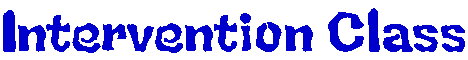Online Activities 11/3/08 - Addition of Decimals, Subtraction of Decimals 11/5/08 - Math Mayhem, Addition of Money, Subtraction of Money 11/10/08 - Disco Dino (level 2-9 or 3-12), Multiplication of Decimals 11/12/08 - Math Magician (level MIXED), Multiplication of Decimals 11/17/08 - Meteor Multiplication, Addition of Fractions 11/19/08 - Hidden Picture, Addition of Fractions 11/24/08 - Multiplication Grand Prix, Addition of Mixed Numbers 12/1/08 - Demolition Division, Subtraction of Fractions 12/3/08 - Drag Race Division, Subtraction of Mixed Numbers 12/8/08 - Number Invaders (multiplication and division), Fraction Frenzy, Multiplication of Fractions 1/12/09 - Space Racer Multiplication, Fraction Frenzy, Multiplication of Fractions by Mixed Numbers 1/26/09 - Math Man, Division of Mixed Numbers 2/2/09 - Math Millionaire, Determining the Ratio 2/9/09 - Sum Sense - Multiplication, Cross Multiplying Proportions 2/23/09 - Multiplication Millionaire, Percents and Fractions, Decimals and Percents, Percents and Decimals 3/2/09 - Sum Sense - Division, Division with Remainders 3/9/09 - Make a Match, Fractions to Percents 3/16/09 - Ordering Integers, Arithemetic 4 (play with a partner; set time to "30 seconds" and select "interget addition", Addition of integers Interactive Math Websites: Math Goodies Hooda Math AAA Math Cool Math A Plus Math Visual Fractions Basketball Math Grid Game (find factors, multiples, primes, triangulr numbers, and/or powers on a grid) Fraction Frenzie Fun Brain Math Brain Math Mayhem (basic addition, subtraction, multiplication, and division) Multiplication.com Virtual Manipulatives Multiplication table practice Math.com Dads Worksheets